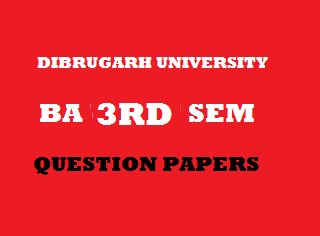## Thursday, January 03, 20192015
(November)
MATHEMATICS
(Major)
Course: 302
(Coordinate Geometry and Algebra - I)
Full Marks: 80
Pass Marks: 32 (Backlog)/24 (2014 onwards)
Time: 3 hours
The figures in the margin indicate full marks for the questions
GROUP – A
(Coordinate Geometry)
SECTION – I
(2-Dimension)
(Marks: 27)

1. (a) Find out the new equation of the parabolawhen the origin in transferred to the point1
(b) Transform, to axes inclined at 450 to the original axes, the equation. 2
(c) Show that the distance between the pointsandis unaltered by translation of axes. 4
Or
Find the angle through which the axes be rotated so that the expressionwill be of the form2. (a) Prove that the equation 4Represents a pair of straight lines inclined to each other at 450.
(b) Find the value of k so that the equation 3may represent a pair of straight lines.
Or
Show that the angle between one of the linesand one of the linesis equal to the angles between the other two lines of the system.
(c) Prove that the product of the perpendiculars from the pointon the lines ofis3
Or
Show that the distance between the point of intersection of the lines represented by the equationand the origin is3. (a) Under which condition does a conic section represent a pair of straight lines? 2
(b) Show that the Cartesian equationrepresents a parabola. 3
Or
Find the diameter of the conicconjugate to the diameter.
(c) Find the condition that the lineis a tangent to the conic 5Or
Reduce the following equation to the standard form:SECTION – II
(3-Dimension)
(Marks: 18)

4. (a) What are the direction cosines of a line equally inclined to the axes? 1
(b) Find the equation of the plane which passes through the pointand is perpendicular to the line joining the pointsand4
(c) Find the equation of the perpendicular from the pointto the lineFind the coordinated of the foot of the perpendicular. 5
Or
Show that the linesare coplanar. Find their point of intersection and the equation of the plane in which they lie.
5. (a) Write down the nature of the non-intersecting lines if their shortest distance be zero. 1
(b) Find the shortest distance between the linesand the z-axis. 2
(c) Find the length and the equations of the shortest distance between the lines5
Or
Find the length of the shortest distance between the linesFind also its equation and points where it intersects the lines.

GROUP – B
(Algebra – I)
(Marks: 35)

6. (a) Subtraction is not a binary composition on the set of natural numbers |N. Justify it. 1
(b) Does the set of all odd integers form a group with respect to addition? 1
(c) Show that if every element of a group G is its own inverse, then G is Abelian. 2
(d) Answer any two of the following questions: 3x2=6
1. Prove that, if and only if G is an Abelian group.
2. Prove that the identity element of a subgroup is the same as that of the group.
3. Find the number of generators of a cyclic group of order 60.
7. Answer any two of the following questions: 5x2=10
1. If for any elements(group), then prove that the equationandhave unique solutions in G.
2. Prove that the product of disjoint cycles is commutative.
3. Prove that a non-empty subset H of a group G is a subgroup G if and only if –
1.2.whereis the inverse of a in G.
8. (a) What is the difference between a complex and a subgroup of a group? 1
(b) Prove that the kernelof a homomorphismofintois a normal subgroup of, whereandare distinct groups. 4
9. Answer any two of the following questions: 5x2=10
1. Ifis a finite group andis a normal subgroup of, then prove that2. Find the regular permutation groups isomorphic to the multiplicative group3. Prove that every subgroup of a cyclic group is cyclic.

***